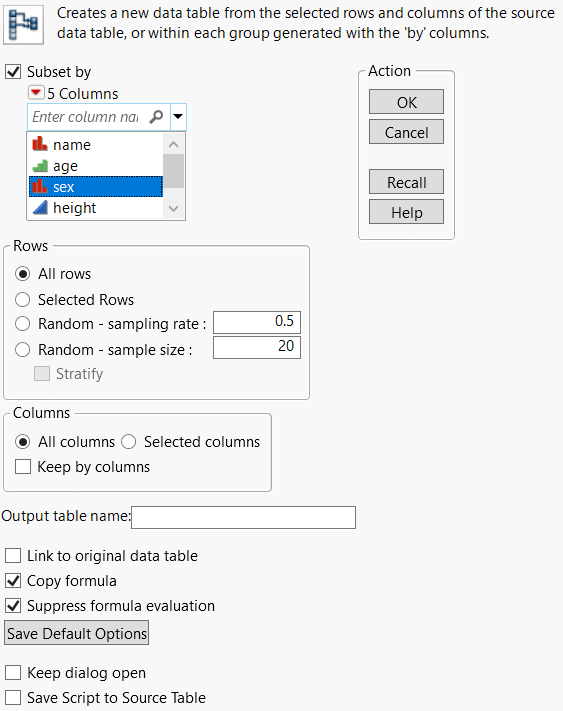Using JMP > Reshape Your Data > Create a Subset Data Table
Publication date: 11/29/2021

# Create a Subset Data Table

You can produce a new data table that is a subset of all rows and columns, only highlighted rows and columns, or randomly selected rows from the active data table.

To create a subset

1. Select Tables > Subset.

Figure 6.2 The Subset Window2. Specify the content that you want to subset. Select any combination of the following:

Subset by (the levels within selected columns)

Rows (all, selected, or random)

Columns (all or selected)

In Figure 6.2, the sex column will be subset.

4. Click OK to create the subset table.

#### Subset Options

Subset by

Subsets by the levels of a column. Select Subset by and then select the columns that you want to categorize for the subset.

Consider the fact that many new data tables might be created. A new data table appears for each level of the column that you specified in the Subset window.

All Rows

Creates a subset table that contains all rows from the active table.

Selected Rows

Creates a subset table that contains only the selected rows from the active table. Selected by default.

Random - sampling rate

Creates a subset table whose data is a random proportion of the active data table. Enter the proportion of the sample that you want in the text box. For example, if you want a random 50% of the data to be included in the new table, enter 0.5 in the text box.

Random - sample size

Creates a subset table whose data is a random sample of the active data table. Enter the size of the sample that you want in the text box. For example, if you want 16 random rows to be included in the new table, enter 16 into the text box.

If you select a random sample that is the entire source table, the result is a random shuffle of the rows of the data table. If you specify columns to stratify, the result is a random shuffle of each of the rows for each group. See Stratified Subsets.

All columns

Creates a subset table that contains all columns from the active table. Selected by default.

Selected columns

Creates a subset table that contains only the selected columns from the active table.

Keep by columns

Retains the column that you subsetted by in the output data tables.

Output table name

Specifies the name of the subset table.

Links the subset table to the original table. When you change values in one table, the other table is updated.

Copy formula

Includes formulas from the original table in the output columns. Include all columns needed for the calculation of the formula. Selected by default.

Suppress formula evaluation

Prevents JMP from evaluating columns’ formulas when the new table is created. Selected by default.

Save Default Options